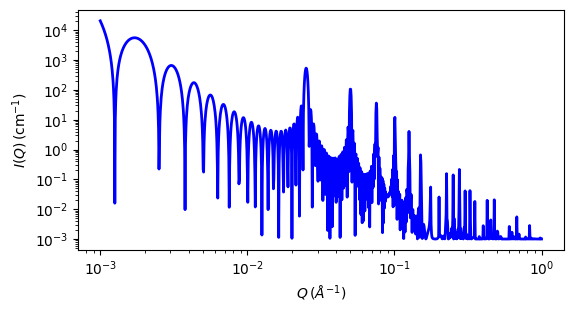# lamellar_stack_paracrystal

Random lamellar sheet with paracrystal structure factor

Parameter

Description

Units

Default value

scale

Scale factor or Volume fraction

None

1

background

Source background

cm-1

0.001

thickness

sheet thickness

33

Nlayers

Number of layers

None

20

d_spacing

lamellar spacing of paracrystal stack

250

sigma_d

Sigma (polydispersity) of the lamellar spacing

0

sld

layer scattering length density

10-6-2

1

sld_solvent

Solvent scattering length density

10-6-2

6.34

The returned value is scaled to units of cm-1 sr-1, absolute scale.

This model calculates the scattering from a stack of repeating lamellar structures. The stacks of lamellae (infinite in lateral dimension) are treated as a paracrystal to account for the repeating spacing. The repeat distance is further characterized by a Gaussian polydispersity. This model can be used for large multilamellar vesicles.

Definition

In the equations below,

• scale is used instead of the mass per area of the bilayer $$\Gamma_m$$ (this corresponds to the volume fraction of the material in the bilayer, not the total excluded volume of the paracrystal),

• sld $$-$$ sld_solvent is the contrast $$\Delta \rho$$,

• thickness is the layer thickness $$t$$,

• Nlayers is the number of layers $$N$$,

• d_spacing is the average distance between adjacent layers $$\langle D \rangle$$, and

• sigma_d is the relative standard deviation of the Gaussian layer distance distribution $$\sigma_D / \langle D \rangle$$.

The scattering intensity $$I(q)$$ is calculated as

$I(q) = 2\pi\Delta\rho^2\Gamma_m\frac{P_\text{bil}(q)}{q^2} Z_N(q)$

The form factor of the bilayer is approximated as the cross section of an infinite, planar bilayer of thickness $$t$$ (compare the equations for the lamellar model).

$P_\text{bil}(q) = \left(\frac{\sin(qt/2)}{qt/2}\right)^2$

$$Z_N(q)$$ describes the interference effects for aggregates consisting of more than one bilayer. The equations used are (3-5) from the Bergstrom reference:

$Z_N(q) = \frac{1 - w^2}{1 + w^2 - 2w \cos(q \langle D \rangle)} + x_N S_N + (1 - x_N) S_{N+1}$

where

$S_N(q) = \frac{a_N}{N}[1 + w^2 - 2 w \cos(q \langle D \rangle)]^2$

and

$\begin{split}a_N &= 4w^2 - 2(w^3 + w) \cos(q \langle D \rangle) \\ &\quad - 4w^{N+2}\cos(Nq \langle D \rangle) + 2 w^{N+3}\cos[(N-1)q \langle D \rangle] + 2w^{N+1}\cos[(N+1)q \langle D \rangle]\end{split}$

for the layer spacing distribution $$w = \exp(-\sigma_D^2 q^2/2)$$.

Non-integer numbers of stacks are calculated as a linear combination of the lower and higher values

$N_L = x_N N + (1 - x_N)(N+1)$

The 2D scattering intensity is the same as 1D, regardless of the orientation of the $$q$$ vector which is defined as

$q = \sqrt{q_x^2 + q_y^2}$Fig. 50 1D plot corresponding to the default parameters of the model.

Source

Reference

1. M Bergstrom, J S Pedersen, P Schurtenberger, S U Egelhaaf, J. Phys. Chem. B, 103 (1999) 9888-9897

Authorship and Verification

• Author: